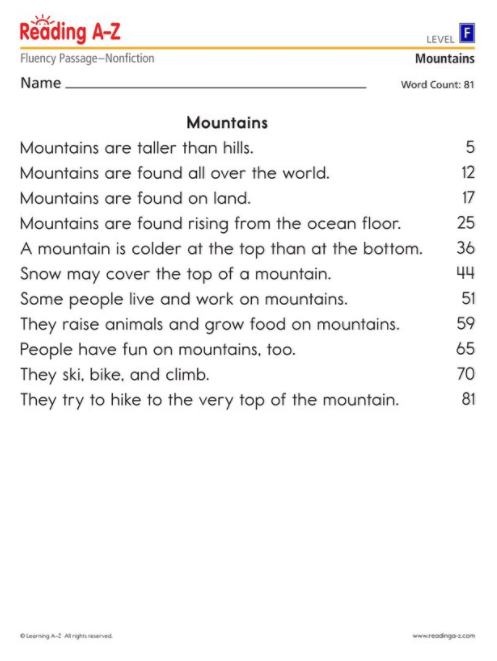Assess reading fluency with two types of assessments. The first type of fluency assessment is a one-minute timed reading of a passage to measure the number and accuracy of words read. There is a fluency passage for each level, F through Z. The second type has a student perform a timed reading of a series of sentences and then answer true/false statements about the sentences to show comprehension. There are three of these assessments, each with more difficult sentences than the preceding one. View our Fluency Standards Table for additional information about recommended reading rates.

## Fluency Assessment Passages

• F
Level F
• G
Level G
• H
Level H
• I
Level I
• J
Level J
• K
Level K
• L
Level L
• M
Level M
• N
Level N
• O
Level O
• P
Level P
• Q
Level Q
• R
Level R
• S
Level S
• T
Level T
• U
Level U
• V
Level V
• W
Level W
• X
Level X
• Y
Level Y
• Z
Level Z